# NET AREA & PERMISSIBLE STRESSES FOR TENSION MEMBERSReading time: 1 minute

#### NET AREA CALCULATION FOR TENSION MEMBERS

When tension members are spliced or connected to a gusset plate by rivets or bolts, some material is removed from the cross-section due to bolt or rivet holes. The net area at any section is equal to the gross area minus the deduction for holes at that section. The deduction for the hole is the product of the hole diameter and the thickness of the material.

Net effective width,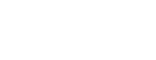Where, s = the staggered pitch, i.e. the distance between any two consecutive rivets, in a zigzag chain measured parallel to the direction of stress in the member,

g = gauge distance

n = number of rivet holes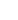= number of zigzags or inclined lines.

This method can be applied to the angles also in which the rows of the rivets in both legs are staggered with respect to each other. For angles, the gross width shall be the sum of the width of the legs less than the thickness. The gauge for holes in opposite legs shall be the sum of gauges from back of angles less the thickness.

#### Net Effective Section for Angles and Tees in Tension

An angle is usually connected to a gusset plate by one leg and a tee is connected through its flange only.Figure: Tension Members

CASE – 1

In the case of single angles in tension, connected by one leg only,Net effective area =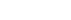Where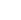is the area of the connected leg,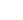= area of the outstanding leg, and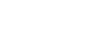For angle sections, a x b x t,Where lug angles are used, the net sectional area of the whole angle member could be considered.

CASE – 2

In the case of pair of angles back to back (or a single tee) in tension connected by only one leg on each angle (or by the flange of a tee) to the same side of the gusset plate,

Net effective area =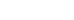Whereis the area of the connected legs (or flange of the tee)= area of the outstanding leg (or web of the tee), and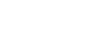CASE – 3

In the case of double angles or tees carrying direct tension, placed back to back and connected to both sides of the gusset,

Net effective area = gross area of section – area of holes

#### PERMISSIBLE STRESSES IN AXIAL TENSION

It is related to the guaranteed minimum yield point of steel with an appropriate factor of safety. Most of the codes assume a factor of safety of 1.67.

The direct stress in axial tension or the effective net area should not exceed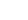given by the equation,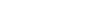Where,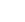= minimum yield stress of steel in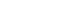(MPa).

Indian Standard IS: 226

It stipulates the following permissible stress in axial tension for steel,

 (i) Plates, angles, tees, I-beams, channels and flats upto and  including 20mm thickness 150 MPa above 20mm thickness to 40mm 144 MPa Over 40 mm thickness 138 MPa (ii) Bars (round, square and hexagonal) Upto and including 20mm diameter 150 MPa Over 20mm diameter 144 MPa

Permissible Combined Stress

When tension members are subjected to both axial loading and bending moment, then the permissible stress is governed by the formula,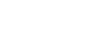Where,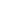= axial tensile stress,= permissible axial stress in tension,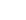= calculated bending stress in tension in the extreme fibre, and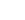= permissible bending stress in tension.